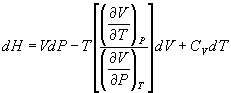5HC - April 1999

Part A (20 x 2 = 40 Marks)

1. What are reversible and irreversible processes?
2. List out any five thermodynamic property changes for an ideal gas
3. Explain the terms 'state function' and 'path function'
4. What is an equation of state? Give the expression for any two equations of state
5. Distinguish between compression and expansion of gases
6. What is vapor-compression refrigeration cycle?
7. What is compressibility factor?
8. Define the term coefficient of performance in a refrigeration process
9. What is a multistage compression?
10. Give the classification of compression processes
11. Give the four Maxwell relations
12. What are the uses of thermodynamic formulations
13. Give any two thermodynamic relations for calculating the entropy change of a real gas
14. Define the term 'phase equilibrium'
15. What are Van Laar and Margules equations?
16. What is a thermodynamic consistency test?
17. Explain the term 'partially miscible system'
18. What are homogeneous and heterogeneous reactions?
19. What is the use of making free energy change calculations in the thermodynamic analysis of chemical reaction equilibria?
20. Give examples of any three industrial reactions
21. Part B (5 x 12 = 60 Marks)

22. (a) Prove that work is a path function, you may take a suitable example for this purpose
23. (b) Give the categorization of systems and processes

Or

24. (a) Explain the limitations of the I law of thermodynamics
25. (b) Give any three statements of the II law of thermodynamics

26. (a) Develop the expression for the work of compression of an ideal gas under adiabatic and non-flow conditions
27. (b) Starting only with definitions and mathematical principles derive the formula

( H/ P)T = V - T( V/ T)P

Or

28. (a) Find the work done when 1 mole of a gas that has the equation of state pv = RT + ATp - Bp, expands in an isothermal reversible process from volume v1 to volume v2. v is the molar volume and A and B are constants characteristic of the gas
29. (b) Briefly discuss about the working of a gas refrigeration cycle

30. Derive the following thermodynamic relations:
31. (a)(b)Or

32. (a) Derive the relations giving the effect of pressure and volume on CP
33. (b) Derive any two Maxwell relations

34. Describe the methods used for testing the thermodynamic consistency of experimentally determined vapor-liquid equilibrium data for binary systems
35. Or

36. The azeotrope of the methanol-benzene system has a composition of 44.8 mole percent ethanol with a boiling point of 68.2oC at 760 mm Hg. At 68.2oC, the vapor pressure of pure benzene is 517 mm Hg and that of ethanol is 506 mm Hg. Calculate the Margules constants for the system and determine the activity coefficients for a solution containing 20 mole percent ethanol
37. (a) Write the expression for the effect of temperature on reaction and equilibrium constant (2)
38. (b) Develop the expression giving the reaction equilibrium constant (K) in terms of the activities of the reactants and products in the case of a homogeneous chemical reaction (10)

Or

39. Calculate the equilibrium percentage conversion of nitrogen to ammonia at 700 K and a pressure of 300 atm. If the gas enters the converter with a composition of 75 mole percent H2 and 25 mole percent N2. The reaction is given by,
40. 1/2 N2 + 3/2 H2 Û NH3

It may be taken that at 300 atm and 700 K,

K = 0.0091 and KV = 0.72 where K is the reaction equilibrium constant and KV is the correction term for fugacity coeffcients.